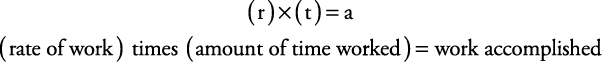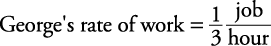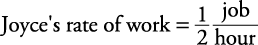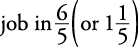## Work

The main formula for work problems isFor example, if a person can do a job in four hours, then his or her rate of work isA person's rate of work is the reciprocal of how long it takes to accomplish the job. Work problems are most easily done in terms of rate of work.

##### Example 1

George can mow a lawn in three hours. Joyce can do it in two hours. If they work together, how long will it take?

George needs three hours to do the job. Therefore,Joyce needs two hours to do the job. Therefore,Let t = the time it takes to mow the lawn together and fill in the chart (see Figure 1).

Together, they accomplish one job. Therefore,Together, they will finish the job inhours, which is the same as one hour and twelve minutes. The check is left to you.